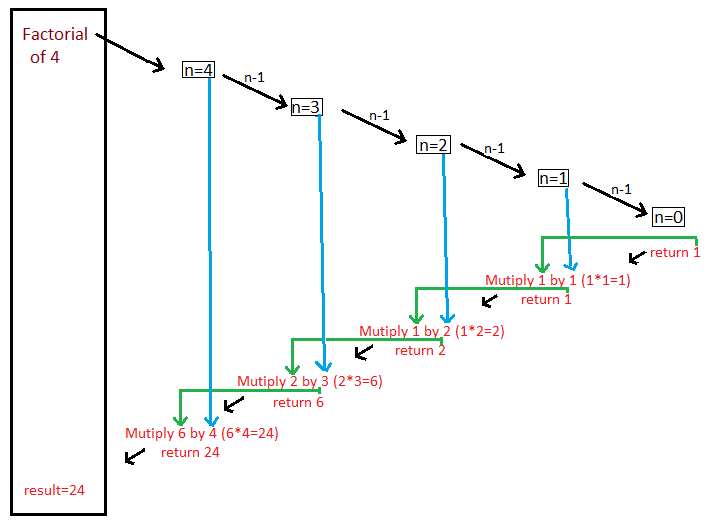### Factorial using recursion in java

In this core java programming tutorial we will write a program to find Factorial using recursion in java

In this program we will find out factorial of number using recursion in java.

input: 4
output: 24

Q.How we caluclate factoria in javal?
A.Let’s say our input is: 4
Than our factorial is 4x3x2x1 = 24.

Q.How we calculate factorial recursively in java?
Let’s understand this by diagram-Initially we start with n=4 and keep on subtracting 1 from n untill n becomes 0, as soon as n becomes 0 return 1. As soon we return 1, because of recursive environment
control will go to calling environment (i.e. when n was equal to 1)
multiply returned result 1 with 1(i.e. n) and return 1.
control will go to calling environment (i.e. when n was equal to 2)
multiply returned result 1 with 2(i.e. n) and return 2
control will go to calling environment (i.e. when n was equal to 3)
multiply returned result 2 with 3(i.e. n) and return 6.
control will go to calling environment (i.e. when n was equal to 4)
multiply returned result 6 with 4(i.e. n) and return 24.

Full Program/SourceCode/ Example to find Factorial using recursion in java >
 /** Copyright (c), AnkitMittal www.JavaMadeSoEasy.com */ public class FactorialRecursionExample {    public static void main(String...args){           int num=4;           System.out.println("Factorial of "+num+" is: "+findFactorail(num));        }       /*    * return factorial of num.    */    public static int findFactorail(int num){                     if(num==0)                  return 1;              return num* findFactorail(num-1);    } } /*OUTPUT Factorial of 4 is: 24 */

Summary >
So in this core java programming tutorial we wrote a program how to find Factorial using recursion in java

Having any doubt? or you you liked the tutorial! Please comment in below section.